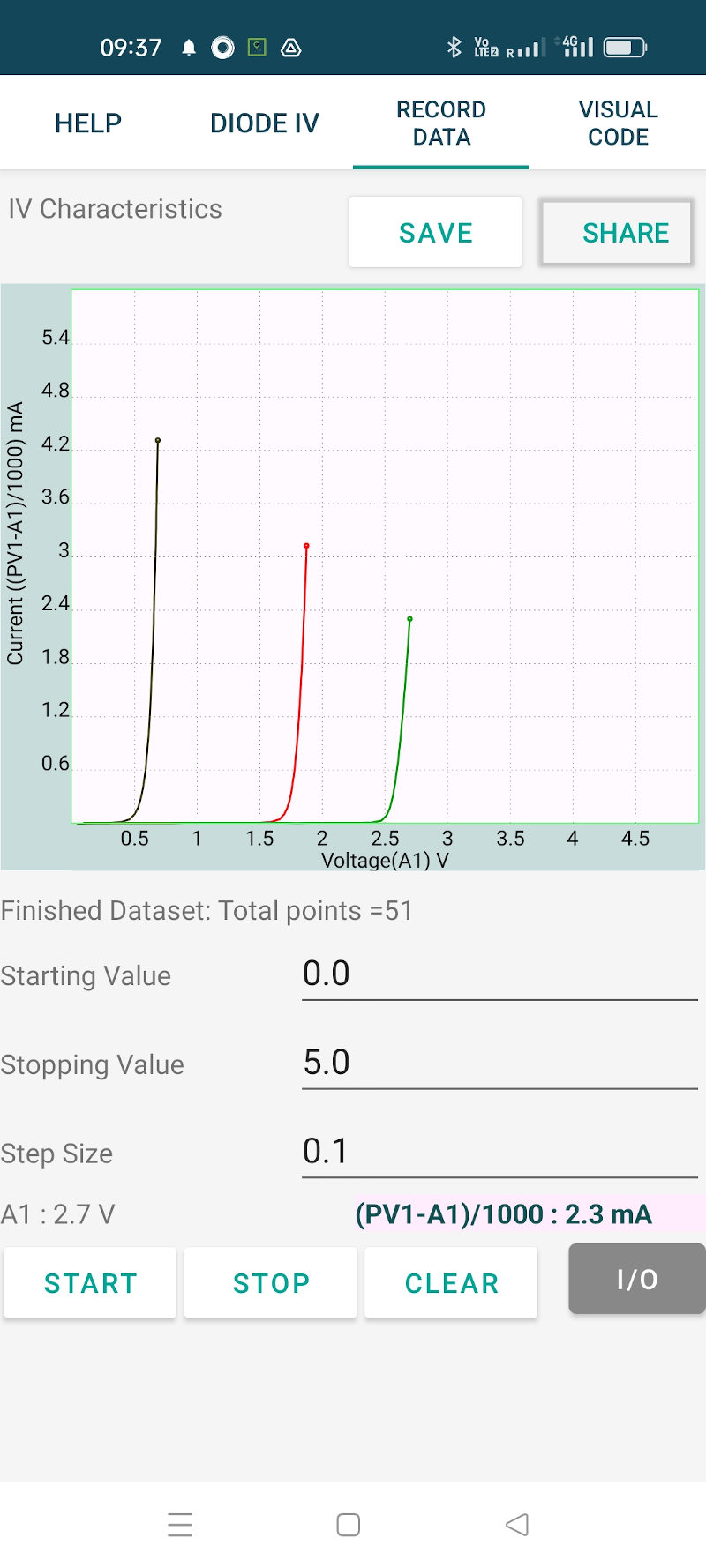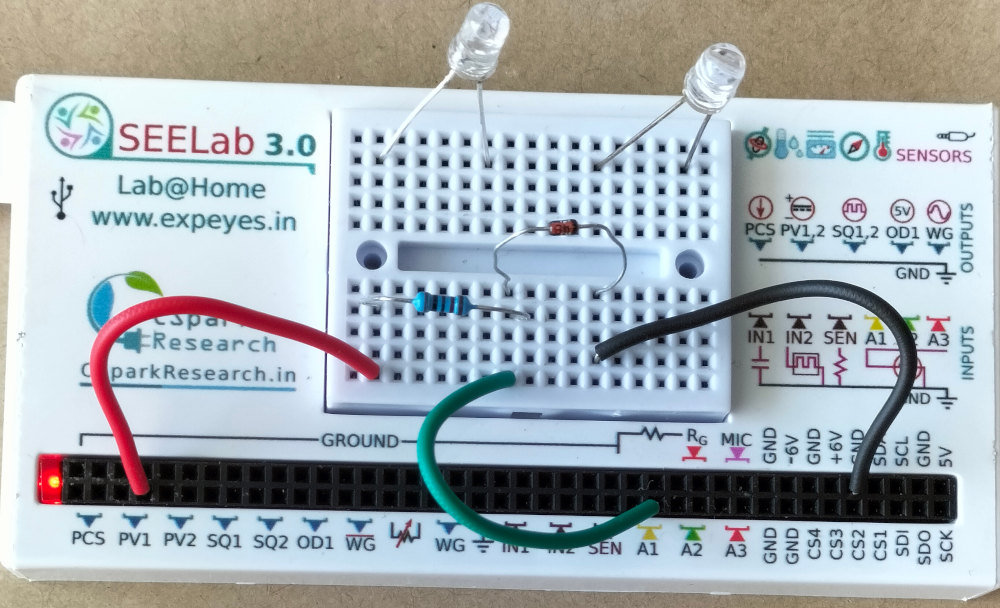# ExpEYES

## VI characteristic of PN junctions

The schematic is wired as shown in the diagram below. The voltage across the diode is measured on A1. The anode of the diode is connected to PV1, through a 1k resistor. Voltage at PV1 is incremented in steps and at each point the voltage across the diode is measured. The current is calculated from i = (PV1-A1)/R. The diode used is 1N4148, silicon diode. The experimental setup and the screenshot are shown below. The results shown are for 1N4148 silicon diode, red and green LEDs.#### Python Code

A Python code may be written to plot the IV graph. The applied voltage is incremented in steps and voltage across he diode is measured. The current is (Vapplied - Vdiode)/Resistance. Python program

import eyes17.eyes
p = eyes17.eyes.open()

R = 1  # in kOhms, gives current in mA
x = []
y = []

v = 0.1
while v < 5:
p.set_pv1(v)
vd = p.get_voltage('A1')
i = (v-vd)/R
x.append(vd)
y.append(i)
print (vd, i)
v += 0.1

import matplotlib.pyplot as plt
plt.plot(x,y, 'x')
plt.show()